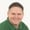FREELessons: 13Length: 1.7 hours• Overview
• Transcript

# 2.2 Creating Objects

Objects are everywhere, so to program in JavaScript we need to understand the basics of creating objects. In this lesson, I'll also introduce the object-oriented programming terminology that I'll use throughout this course.

## 6.Conclusion1 lesson, 00:43

### 2.2 Creating Objects

In this lesson, we are going to look at the basics of creating objects. Now I'm aware that this is review for probably most of you, but I am going to introduce some of the terminology that I'm going to use throughout this course. So there's that and plus, there's nothing wrong with review. So let's just get started by writing the code that we use to write. Whenever we created an object, we would do so using the object constructor and then we would populate to the properties and methods one at a time on each statement. So we would have obj.firstName and lets just assign that to john. And for each property, we would have a line of code with object.property equals and then the value. And when it came time for a method, we would do the same thing. We would have object.property and then assign that to a function. However, in most cases, our function was actually defined instead of using an anonymous function, because we didn't use a lot of anonymous functions back then. So, it would look more like this. Now, I use the term property for the greet property and that's because it is a property. JavaScript, technically does not have methods. It has properties that contain function objects and that is very clear by what you see on line eight. We are creating a property and assigning it to a function. So throughout this course, I am going to refer to methods either as a method or as a property. Both are correct. One is logically correct, method. The other is technically correct, property. I might say that this object has two properties. I might say that it has one property and one method and I might say that it has two members. Members are public properties and methods of an object. So, I can use a variety of terms to define the properties and or methods of an object. It just really depends upon how I'm feeling at that particular point in time. Now the way that we created objects evolved over time. Instead of using the object constructor, we would then use literal notation to create that object. That would save us some keystrokes and of course, it would also save us in file size, but we would still have code that would look like this. We would create our object first and then we would define the properties and methods one at the time later on, but then we started to change from that as well. It made more sense to define everything using object literal notation. For one reason, it saves us time, because it's less typing, it's less repetition. Because we don't have to say, object.property equals value and then object literals also execute faster than creating the object. And then creating the properties one at a time, because JavaScript can execute a single complex statement faster than multiple simple statements. So instead, it would look like this. We would define our property followed by a colon. We don't use an equal sign in object literal notation and then the value, so john and then we would separate each property definition with a comma. So we have firstName: "john", and then greet. And in this case, we are going to use an anonymous function, because that's typically what we do now. So whenever we create an object, this is what it looks like. It's nice and concise. It's all grouped together, so we know that everything inside of here is part of this object. Now the problem with creating objects individually one at a time is if you need multiple objects with the same interface, then you have to create those objects. Now I use the term interface there and throughout this course, I'm going to use that to refer to the shape of an object. So the interface of this object is that it has a first name property, which is a string and then it has a greet property, which contains a method. Now I might also say, shape. The shape of this object is going to be the same as the shape of another object that I'm just going to copy and paste and then we can change the value of first name. So we could say that these two objects have the same interface or these two objects have the same shape. Now this particular problem of creating multiple objects with the same shape, really isn't a problem at all, because we can do so in a variety of different ways. The first way is to create them using the factory pattern, which is using a function to create our objects, which is what we are going to look at in the next lesson.

Back to the top